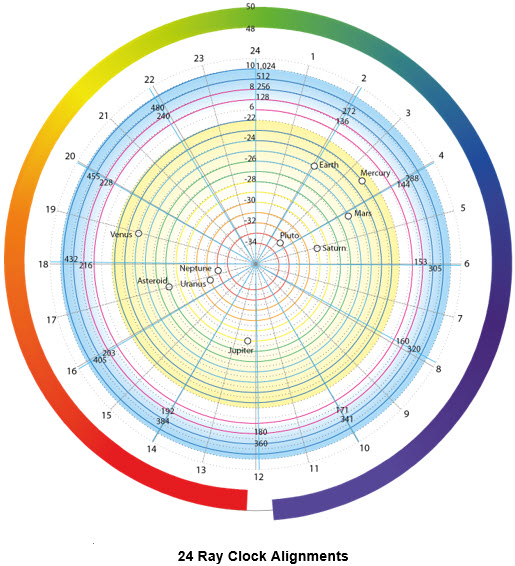# Spirals of Harmony

Harmony is a key element in structure.  It is of fundamental importance to the structure of sound frequencies in music, color in art, proportions in architecture, etc.  Sound provides an excellent basis for exploring the nature of harmony.

We perceive sound in terms of frequency or pitch.  This is measured in terms of cycles of variation per second – the higher the frequency the more cycles per second.  Our perception of frequency is proportional.  The steps in frequency get bigger as we go up the frequency scale.  We perceive the difference between a note and another at half its frequency as the same as that between the note and double the frequency.  For example the difference between notes at 100 and 50 is perceived the same as that between 200 and 100.  The numbers relate to cycles per second also called Hertz (Hz).

This proportional variation differs from linear patterns of change, e.g. 50 : 100 : 150, where the steps are equal.  Linear patterns are like the steps of a stairs, where the increments are uniform and add one after another.  With proportional patterns the steps are multiples of the current position – so the higher the frequency the bigger the steps.  This process leads to repeated multiplication also known as exponentiation.  This is the pattern for exponential growth.  Such growth lends itself to spiral formation.

References to the Krystal Spiral are based on the original definition of this spiral translated by E’Asha Ashayana from Eieyani sources.  This is gratefully acknowledged.  More detailed information is provided in –  Spirals of Creation.  The active guidance, support and assistance of ASaN Deane and Wilbur contributed immensely to the development of this information and is also gratefully acknowledged.  Likewise, the Kathara Team provided immense support and facilitation.

## Octaves

The structure of music and of sound generally is determined by frequency, which in turn depends on number.  Harmony in music is determined by simple ratios between these numbers.  The simplest possible ratio is 2 : 1.  We perceive greatest harmony or resonance between a note and a note at double its frequency.  This doubling of frequency provides a unit of harmonic structure.  The band of frequencies between a note and another at double the frequency is defined as an octave.  Musical notes or tones are therefore divided into a sequence of octave bands.  Each octave doubles the frequencies of the previous octave band and in turn is doubled by the next octave above it.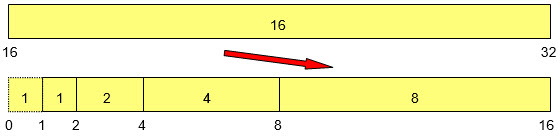This process is illustrated in the diagram above.  This is a simple sequence of octave bands. The end of each band is double the beginning and each band is double the length of the previous one.  The initial band between 0 and 1 isn’t a band of itself but rather the sum total of an infinitely large collection of progressively smaller bands below 1 heading towards 0.

We can see that each band is equivalent to the sum total of all the previous bands.  This is illustrated for example by band 16 overlaying the collection of all prior bands in the diagram above.  This is true for all such octave bands.

Because of the uniform doubling of frequency every note in an octave band will be double the frequency of the note in a similar position in the octave below.  For example a note in the middle of octave 8 will be double the note in the middle of octave 4.  This provides maximum harmony or resonance between such notes.

The next task is to sub divide each octave into a collection of individual notes.  The spacing of these notes must also be proportional like the sizing of the octaves themselves to provide a smooth progression up the scale from one octave to the next.  They should therefore be spaced by uniform multipliers.  Within an octave the end note being twice the starting note will have maximal harmony with it.  Likewise for the intermediate notes harmony is enhanced when these have the simplest numerical ratio with the starting note consistent with uniform spacing.  So there is a balance to be achieved between uniform spacing and simplicity of numerical ratio with the starting note.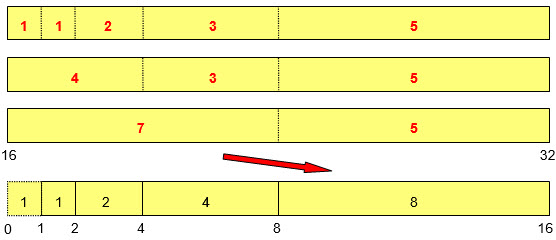The optimal balance is achieved by sub dividing octaves by 12.  This generates 12 notes in each octave with each note resonating with equivalent notes in neighbouring octaves.  Uniform spacing is achieved by 12 equal steps of multiplication between 1 and 2.  What number multiplied by itself 12 times would give 2?  This is the 12th root of 2  =  21/12  =  1.0595…  Multiplying the starting frequency repeatedly by this number will space out 12 notes within the octave band.  This ensures proportional spacing.  However these notes while generally close to are not in direct simple numerical ratio with the starting frequency.  Harmony is enhanced by deviating slightly to pick up the nearest simple ratio.

The outline division of an octave into 12 notes is illustrated in the diagram above.  Because the notes are proportionately spaced there will be more notes at the lower end of the octave than the higher end.  The twelve-fold division produces 7 notes in the lower half and 5 in the upper half of the octave, as illustrated in the second band from the bottom in the diagram above.  The 7th note bisects the octave.  The red figures indicate numbers of notes and not band width.  Likewise the lower half octave sub divides approximately with 4 notes in the first and 3 in the second quarter, as in the third band from the bottom above.  There is further sub-division approximately as indicated in the topmost band.  So this 12 fold division also picks up some of the structure of the earlier octaves.

The simple octave numbers in the diagrams above are the same as the Krystal Spiral number sequence –

1, 2, 4, 8, 16, 32, 64, 128, 256, 512, 1024, etc.

## Octave Spirals

These numbers plus the exponential growth indicate a spiral formation.  This can be used to explore the structure inherent to musical harmony.

The harmonic pattern repeats every octave.  So we can ascribe a complete spiral cycle to each octave.  This is illustrated in the diagram over.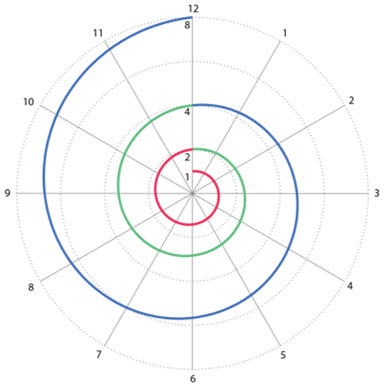The spiral combines proportional or exponential expansion with uniform rotation or circular motion.  Circular motion is always associated with frequency – a cycle corresponds to a complete rotation of a circle at some level.  So it is very appropriate to be relating frequency to a diagram with a circular element.  The radial distance from the centre is proportional to and measures the frequency, while the angular distance around the circle measures relationship.

The first octave shown in red expands from 1 to 2 as the spiral rotates around the circle.  The next in green goes from 2 to 4 and so on.  Similar octave spirals expand outwards through 8, 16, 32, etc. in ever increasing and infinite magnitude.  Similarly inner octave spirals contract inwards from 1 on an ever smaller scale approaching zero.

The spiral circle is divided into 12 sections corresponding to the twelve fold division of an octave.  These are illustrated by the 12 radial lines in grey.  These are aligned in clock formation with the starting point vertically upwards in the 12 position, because of our familiarity with the clock.  The spirals are also set rotating clockwise.  The spirals rotate uniformly around the clock so that the 12 sectors can be spaced evenly.  The outward (radial) motion of the spirals is proportional with the steps increasing from each sector and octave to the next.  The spiral diagram is an accurate representation of the formation of octaves and of their twelve fold division.

The rays or radii indicate relationship between the octaves.  As we move out on any ray the notes that we meet on each spiral double in frequency as we move through the octaves.  All the notes on the same ray therefore have maximum harmonic relationship.  They resonate with each other.  The rays therefore quickly and easily identify resonance.

We can expand the octave spirals out further into a range of frequencies of greater interest for musical scales, as illustrated in the diagram over.  The inner spirals are still there, but are not drawn for clarity.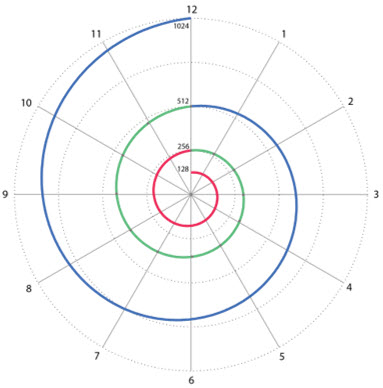## Conventional Music Scales

The frequencies for notes in some of the main conventional music scales are summarised in the table below.  The octave spanning notes C4 – C5 is taken as the primary reference.  The 12 notes are indicated with their corresponding frequencies in the main scales.  Note A is taken as a reference for tuning.  This is tuned conventionally to 440Hz.  This in turn sets the frequencies for the other notes using the proportional ratios developed for each scale.  All higher scales are doubles or repeated doubles of these frequencies, while lower scales successively halve these frequency values.

The Equal Temperament scale assigns the notes in exact proportional alignment, i.e. these notes are uniformly spaced on the spiral diagram above.  The Pythagorean scale deviates slightly from proportional spacing to pick up more harmonious numerical proportion with the starting frequency.  The numerical ratios for the Pythagorean scale are indicated in the second table below.

The Just Intonation scale only uses 7 notes within each octave.  However these are spaced on sub division units of 12 similar to the Pythagorean scale as we can see from the correspondence of frequencies.  There are gaps corresponding to missing notes.  Again the ratios for this scale are indicated in the table over.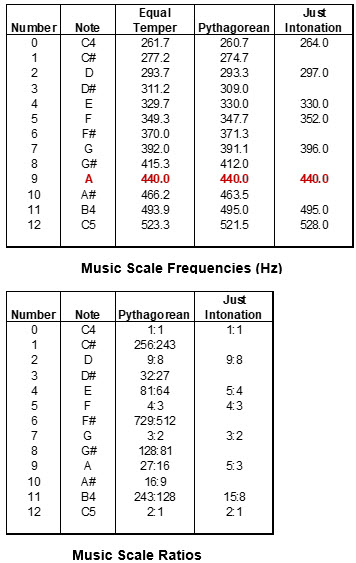The Pythagorean ratios may look odd but there is a definite structure to them.  They are formed from ratios between two number sequences as illustrated in the diagram below.  We have one sequence formed from powers of 2 (1, 2, 4, 8, 16, … )  This is shown along the left side of the diagram. This corresponds with the Octave or Krystal Spiral number sequence.  Along the right side of the diagram we have powers of 3 (1, 3, 9, 27, 81, … ).  These correspond with the Merkaba Spiral number sequence.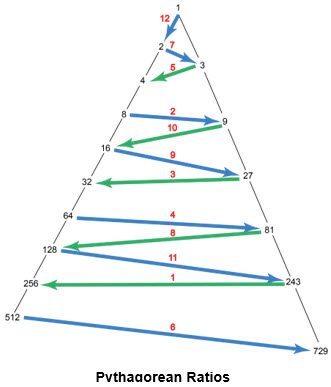The Pythagorean ratios are formed between numbers from each sequence or side of this diagram.  The ratios are indicated by arrows linking the numbers in question with the arrow pointing towards the numerator or top number in the fraction.  The red numbers associated with each arrow indicate the note number matching that ratio.  We can see that all the numbers are included and that there is a definite progression to the ratios.  It should be noted that these ratios only include 2 and 3 number elements.  The number 5 is excluded.  2, 3 and 5 form the key prime numbers and together are fundamental to most number formation.  When 5 is included in the Just Intonation sequence of ratios above we get simpler proportions, if slightly less accurate in some cases.

## Enhanced Music Scales

Looking at the numbers in the scales above we see that the octaves don’t align on natural octave boundary numbers, e.g. 256, 512, etc.  However it is interesting to note that the scales start with a note C that is relatively close to a natural octave boundary.  If we modify this note to align with a natural octave boundary we get the results indicated in the table over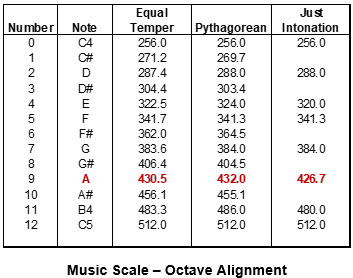Here we get the very interesting result that the tuning reference note A moves to 432 in the Pythagorean scale.  This frequency is discussed quite a bit in avant garde music circles.  It has the very significant benefit of aligning octaves in the Pythagorean scale with natural boundaries.  We also see that the Pythagorean numbers are much tidier and more interesting than in the conventional scales above.

In addition to the proportional spacing and harmonic proportion requirements mentioned above we can introduce an additional requirement that the numbers for note frequencies align with Krystic number patterns.  We can introduce a modified scale to additionally meet this latter criterion.

The Pythagorean ratios and associated numbers are built up from the numbers 2 and 3.  There is no provision for the other key prime – 5.  It is desirable to include this in the number ratios also.

## Krystal Music Scale

Looking at the mid-way point on the Equal Temper scale we see that note 6 has a frequency of 362.  This is very close to a key number in cosmic structure – 360.  360 in fact is closer to the accurate proportionately spaced point than its Pythagorean equivalent – 364.5.  This is a very fortuitous ‘accident’.  We include this value in a proposed Krystal sequence of musical notes in the table over.  360 is a powerful number with a lot of structure.  It is used as the basis for dividing a circle into 360 degrees.  The number of days in a year also approximates to this.  360 = 24 x 15.   Breaking it down into its prime factors we get 2.2.2.3.3.5, where the dot indicates multiplication.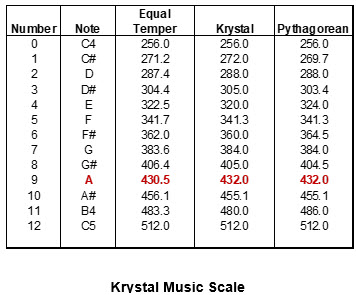This mid-point (6) in the octave has an exact frequency √2 = 1.414… times the fundamental or starting octave frequency.  The Pythagoreans sought a numerical ratio for this critical √2 number.  They were dismayed to find there is no simple or integer ratio for this.  It’s very interesting that the circular number 360 comes up as an excellent approximation in relation with 256.  √2 being the diagonal ratio for a square is associated with the square.  So we have an interesting resonance here with the old problem of squaring the circle!

Once we establish 360 on the 6 o’clock ray inner octaves will pick up the numbers 45, 90, 180 successively on this ray, while outer octaves will hit 720, 1440, etc.  These are illustrated on the octave spiral diagram below.

So we have the Krystal Spiral number sequence expanding out along the 12 ray.  We have the circle number sequence with its associated divisions and multiples (45, 90, 180, 360, 720, etc.) expanding along the 6 ray.  It is interesting that these two opposed rays carry the exponential expansion sequence at one end and the circular rotation numbers at the other.  These encompass the two aspects of a spiral.

The grey ray line at the 6 position shows the exact location for proportional spacing.  The light blue line slightly to the right shows the ray position to pick up the 360 number sequence.  The deviation or ‘error’ is relatively small.  12 and 6 are primary ray positions on the clock diagram.

7 is also an important ray.  This has a simple and powerful numerical ratio of 3:2 with the fundamental frequency.  This ray picks up the numbers 3, 6, 12, 24, 48, 96, 192, etc.  It picks up the important 12 number sequence.  Again the grey ray shows the exact 7 position and the blue ray that associated with the 3:2 ratio.  In this case there is negligible error.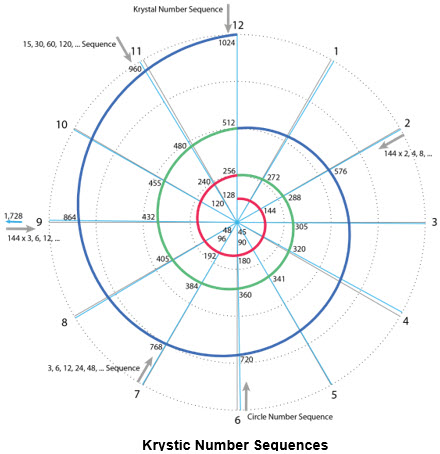The 3:2 ratio gives a value of 1.5, i.e. it divides the octave frequency range in two.  The 7 ray aligns with the mid frequency in each octave.  We can see this also from the numbers, e.g. (256 + 512) / 2 = 384.  This means that we have 7 notes in the lower half of each octave and 5 in the higher half, i.e. each octave has 7 lower and 5 higher notes.  This also means that when we overlay an octave on the full sequence of earlier octaves as in the second diagram above the 7 note will align pretty accurately with the starting point of the prior octave.  It will align with the prior octave boundary.  This creates a strong resonance effect with prior octaves and enhances harmony thereby.

In the 11 ray position we have chosen the Just Intonation ratio of 15:8.  This is a simpler and much more powerful ratio that the 243:128 values used in the Pythagorean scale.  It is only slightly less accurate than the Pythagorean ratio.  However it picks up 15 exactly and the numbers that grow out from this – 30, 60, 120, 240, 480, etc.  This is the 15 number sequence.  Again the blue ray indicates the degree of deviation from exact proportional spacing.

Another important Krystic number is 144 (12 x 12).  This number is picked up on ray 2.  On an inner octave we have the number 9.  This then expands outwards – 18, 36, 72, 144, 288, etc.  Here we have the powers of 2 expansion of 144, i.e. x2, x4, x8, etc.  We also pick up the triple or x3 multiples of 144 on ray 9.  Here we have 432

(144 x 3), 864 (144 x 6), 1,728 (144 x 12), etc.  So the key 144 numbers are distributed between rays 2 and 9.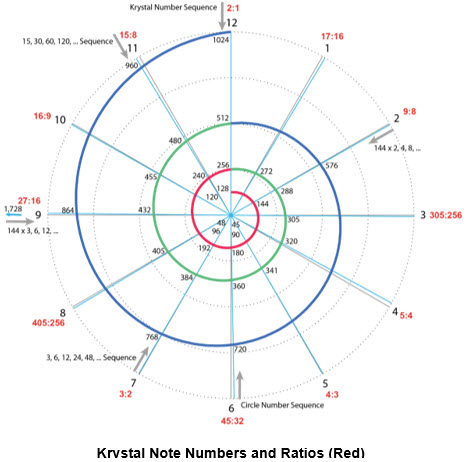It can be noted that where two notes are 7 rays apart their frequencies will be very close to a ratio of 3:2.  Similarly where two notes are 9 rays apart their frequencies will be close to a ratio of 5:3.  This 5:3 ratio is exact between the notes on rays 11 and 2, e.g. 480 / 288 = 5 / 3 = 12/3.  This is an important Krystic ratio particularly for Krystic Merkaba.

This pattern therefore embraces all key Krystic numbers.  Octave division by 12 is very powerful as this is the only number that picks up so many resonance alignments.  Also correct alignment of the octave boundaries is key to picking up all the important Krystic numbers.  This is the only pattern that will achieve it.

We next fill in the frequency numbers for the remaining rays.  These are illustrated in the diagram above.  This also includes the ratios or proportions associated with each ray on the octave spirals.  These are marked in red around the perimeter.

On ray 4 we use the important ratio 5:4.  This differs from the Pythagorean ratio of 81:64 but aligns with that for the Just Intonation scale.  It is only slightly less accurate than the Pythagorean value.  However the 5:4 ratio is more powerful.  Critically it brings the number 5 into the structure.  In the 4 – 8 octave applying the 5:4 ratio brings 5 in directly as the 4th note in that octave.  This then expands along the 4 ray as 10, 20, 40, 80, 160, 320, etc.  Additionally 5/4 = 1.25.  This marks the boundary for the quarter point in the octave, just like 3/2 = 1.5 marks the half way point.  So when we overlay scales as in the second diagram near the start of the article this note will align with the boundary for the second prior octave.  This enhances harmonic strength.  This means that there are 4 notes in the first quarter of an octave, 3 in the second quarter and 5 in the higher half octave.

For ray 1 we have the proportionately spaced Equal Temper value of 271.2.  This tone is very close to the ratio of 17:16 = 136:128, which would give a value of 272.  The significance of 17:16 is that it marks the 1/16th point in an octave.  When we overlay an octave on its prior values this 17:16 point will aligns exactly with the boundary for the 4th prior octave.  Furthermore as we shall see further on the 4th prior octave carries Krystal Spiral resonance in addition to octave resonance.  It carries super resonance.  This provides powerful harmonic relationship for the first ray line.  The ray is also very close to the quadrant number of 270, i.e. the third quadrant (3 x 90) in a circle.  This would give a ratio of 135:128.  However 272 carries more powerful resonance and is taken as the number here. This is more accurate than the Pythagorean ratio of 256:243, which gives a value of 269.7.

Similarly on the 2 ray we have the ratio 9:8.  This marks the 1/8th point in an octave.  This point aligns exactly with the boundary of the third prior octave when we overlay them.  We therefore have 7 notes in the first half of an octave, 4 in the first quarter, 2 in the first eighth and 1 in the first sixteenth, as illustrated in the second diagram above.  The 12 note division of an octave uniquely supports such alignment with prior octaves.  This enhances harmonic strength.

On ray 3 we have the Equal Temper value of 304.4.  This is very close to 305.  This in turn is 3 times the magnetic spin speed for Quatra phase Merkaba (1012/3).  It is also in the correct 3 position for magnetic Merkaba on the clock diagrams.  So 305 is taken as the number value for the 3 ray.  This leads to a ratio of 305:256.  This is less simple than the corresponding Pythagorean ratio of 32:27.  But it is more accurate.

The 3:2 ratio for the 7 ray mathematically leads to a 4:3 ratio for the 5 ray.  This is an important ratio and is highly accurate for this ray.  The numbers on this ray don’t appear to have inherent significance.  But they do carry this important proportion.

On the 8 ray we have an Equal Temper value of 406.4.  This is very close to the octant number of 405 (9 x 45).  45 is an eighth division of the 360 value for a circle.  It forms an octant just as 90 forms a quadrant.  405 is also the number for Trans Harmonic Quatra Phase Merkaba.  405 is taken as the number for this ray.  This leads to a ratio of 405:256.  Again this is less simple than the Pythagorean ratio of 128:81, but is more accurate.Finally for ray 10 we use the Pythagorean ratio of 16:9.  This is related mathematically to the 9:8 ratio for ray 2.  It also has resonance with the 4:3 ratio for ray 5 – it is the square of this ratio.  It aligns pretty accurately with the proportionately spaced value – 455.1 vs 456.1.  The numbers in this sequence don’t appear to have intrinsic significance.  The values are close to 450, which is a quadrant number (5 x 90).  However the error in adopting this would be significant, i.e. greater than 1%.

The proposed Krystal music scale is therefore summarised in the diagram above.  This shows a single octave.  Other octaves would be exactly double or half these values, as summarised in the table below.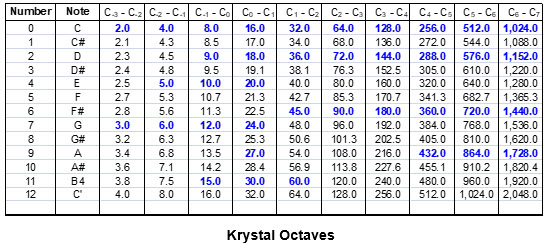Some of the numbers of significance are highlighted in blue.  The basic numbers 1, 2, 3, 4, 5, 6, 8, 9, 10, 12, 15, etc. are all included.  1 is in an earlier octave not shown in the table.  It should be noted that prime numbers are not included unless they are explicitly entered into the structure.  The numbers in the scales are formed from ratios based on powers of 2, i.e. they depend on other numbers.  Prime numbers are not built from other numbers.  Each is unique – hence the term prime.  They won’t arise in a structure like this unless they are built-in.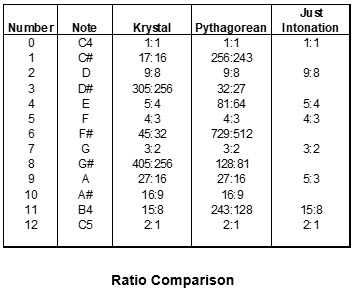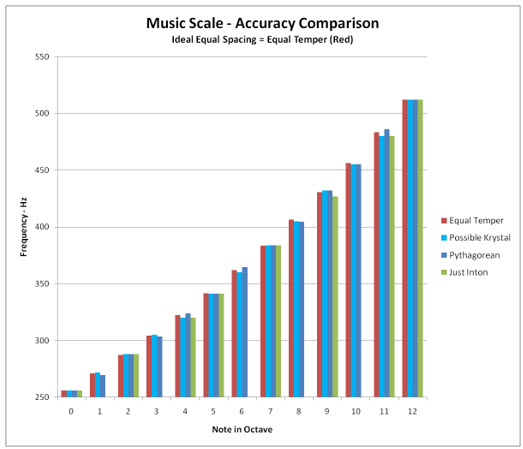The key primes 2, 3 and 5 and multiples of these arise because they are structured into the ratios.  5 or any numbers based on it wouldn’t arise if 5 weren’t incorporated in the ratios above.  Hence the significance of including it explicitly.  The Pythagorean ratios exclude it.  The remaining primes up to 12, i.e. 7 and 11, are not included.  Likewise the remaining prime up to 15, i.e. 13, is not included.

The graph above compares the relative accuracy of the scales.  The red set of bars is the Equal Temper scale with exactly proportionately spaced notes.  The light blue bars represent the proposed Krystal scale, while the darker blue are for the Pythagorean scale.  Except for notes 4 and 11 the Krystal scale is more accurate.

## Cosmic Harmony

We now have a clean and powerful conceptual framework for studying harmonic relationship.  Any frequency is associated with circular motion at some level.  The cycles correspond to rotations around a circle.  The frequency measures the number of such cycles in a unit of time.  If we take a consistent unit of time, such as a second, we can bring the frequency numbers into relationship on a consistent basis.  It doesn’t matter what the time unit is so long as it is used consistently.

The spiral diagram captures the circular motion in addition to the exponential expansion of the numbers.  The harmony resides in the circular relationships between these numbers.  Once we establish the correct spiral structure the circular alignments will reveal harmonic resonance.  The octave spiral with its twelve fold division in terms of clock rays is a powerful tool both for illustrating and analysing such relationship.  It is also relatively easy to use in practice.

We can take any frequency (f) measured on a consistent basis of cycles per second (Hz).  Then take the logarithm of this number to base 2.  This is easily done in excel for example using the standard function – Log (f, 2).  The whole number portion of this result gives us the octave number in question.  The decimal or fraction part tells us where in the octave cycle it arises.  Take the 360Hz note for example.  Log (360, 2) = 8.492…  This tells us that this frequency arises in the 8th octave out (starting from 1 – 2 as octave 0).  The decimal part (0.492…) tells us that it is 49.2% of the way around this octave circle, i.e. close to ray 6.  If we multiply this decimal part by 360 we can plot the degree position on the spiral exactly.

All frequency whether sound, light, planetary motion, dimensional frequency bands, etc. can be related on this basis.

Cousto [Ref 1] has already done excellent work in ascribing frequencies to the planets and in using octave analysis to bring these into relationship with corresponding sound and light frequencies.  We can use the octave spirals to illustrate these relationships more explicitly and discern additional structure.

We are dealing with both very large and very small number values here.  These span many octaves.  Using linear radial scales for the outward expansion we can only show 3 or 4 octaves clearly on a single diagram.  We can develop the diagram further by using a logarithmic scale for the radial expansion.  This handles a much greater range of numbers.  The spirals are evenly spaced inwards and outwards from octave to octave.  They are also more uniform within an octave on such a scale.  This doesn’t in any way affect the circular or harmonic relationship between frequencies.  It just simplifies or clarifies the diagrams so that they can handle large number ranges.

## Krystal Spiral Harmony

In octave analysis a complete cycle corresponds with expansion by 2.  This is related to the Krystal Spiral.  However in the case of the Krystal Spiral each complete cycle or rotation of 360° corresponds to expansion by 16.  The Krystal Spiral expands by 2 in every quadrant of 90°.  So a Krystal Spiral cycle spans 4 octave cycles.  These are illustrated in the diagram for the Krystal Spiral below.

In this diagram 2 cycles of spiral expansion are shown – first cycle in red and the second in blue.  It can be seen that the spiral is expanding much faster than the octave spirals above.  However there is direct relationship between the two types of spiral.  Every quadrant on the Krystal Spiral corresponds to a complete octave spiral cycle.  The octave cycles are illustrated on the Krystal Spiral using the encircled numbers.  The Krystal Spiral has its twelve fold clock division illustrated.  This is further sub divided by 4 to align with the corresponding division of the octave cycles.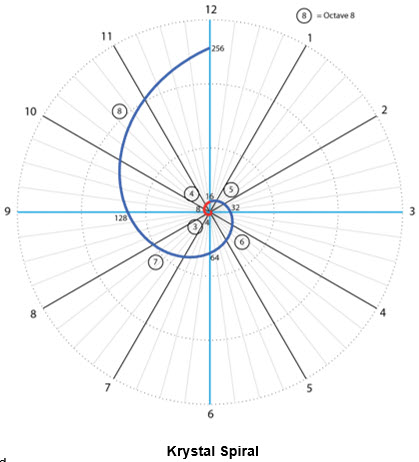We can see from this diagram that where we have Krystal Spiral alignment along the rays we also have octave alignment with the cycles spaced 4 octaves apart.  For example octaves 4 – 8, 3 – 7, etc.  Thus where we have octave alignment with the octaves spaced 4 cycles apart we also have Krystal Spiral alignment.  We have both octave and Krystal Spiral resonance.  We can refer to this condition as super-resonance.

We can therefore also use the octave charts to discern Krystal Spiral resonance.  Just look for 4 octave spacing in addition to octave resonance.  If we were to use charts based on the Krystal Spiral directly it would be harder to discern the octave resonance between the quadrants.  The octave spiral charts meet both analyses most conveniently.

## Planetary Harmony

Cousto  (The Cosmic Octave – Origin of Harmony.  Cousto.  Life Rhythm 2000)  has assigned natural frequencies to the planets along the following lines.  The basic cycle is an orbit around the sun.  The time for each planet in the solar system to complete such an orbit around the sun is summarised in the table below.  The Sidereal Period is the time taken to complete an orbit using the fixed stars as reference.  The time is measured in earth years.  This time period can be converted to seconds to give the number of seconds needed to complete a cycle.  Inverting this number gives the portion of a cycle completed by the planet in a second.  This is a natural frequency associated with the planet.  The number of course is very small.  This approach is both valid and reasonable.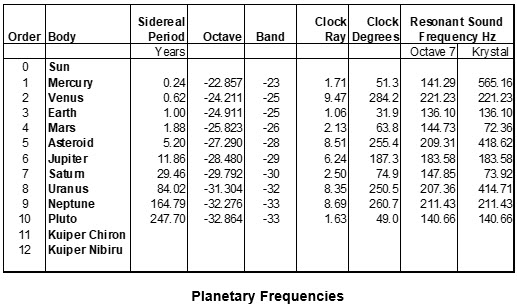The planetary frequencies can then be converted to Octaves.  Because of the very small numbers in question these have a negative value as shown in the table.  We can graph these planetary frequencies on the octave spiral chart as illustrated in the yellow portion of the diagram below.  The spread of planets spans some 10 octave cycles from about -33 to -23.  The octave numbers are shown to the left along the 12 ray line.  So we need to compress many spiral cycles to fit in a compact chart.  The planetary frequencies are distributed among the clock rays as shown.  The distribution is mathematically accurate.

This gives a good indication of the spread of frequencies and of their relative alignments.  Note that the more distant planets have longer orbits and slower frequencies.  Their numbers are progressively closer to zero.  Hence they arise in the inner bands.  There are infinitely many inner bands approaching zero. The center portion of this formulation of the diagram doesn’t give a good feel for this, but is accurate nonetheless.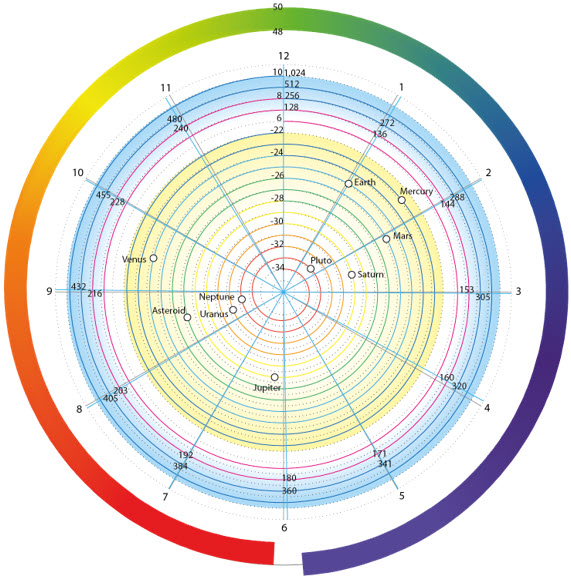Further out in the blue section of the chart the sound frequencies associated with the music scale mentioned above are summarised.  There is a gap of some 28 octave bands between the planetary frequencies in yellow and the sound ones in blue.  This is compressed in the diagram.  But this does not affect the accuracy of the alignments.  Four sound octave cycles are illustrated in the blue section.  These run from octave 6 (26 = 64) out to octave 10 (210 = 1,024).  The number values for each ray are indicated for the middle two octaves only.  The other octave numbers are either double or half these values.

This gives an indication of the resonant alignment between planetary frequencies and sound tones.  It also gives an indication of the accuracy of alignment with specific music notes.  The specific frequencies associated with each planet in octave 7, i.e. between 128 and 256, are summarised in the table above.  These are the frequencies assigned by Cousto.  We can additionally look for Krystal Spiral alignment by moving out through the chart in multiples of 4 octaves.  This gives us a set of sound frequencies that have both octave and Krystal Spiral resonance with the planets.  These are summarised in the rightmost column of the table above.

It can be noted that the earth frequency aligns pretty accurately with the ray 1 sound tones.  The earth frequency holds both octave and Krystal Spiral resonance with the sound tone at 136.1Hz.  The corresponding ray 1 tone is 136Hz!  The ray 1 tones in turn have a strong Krystal Spiral resonance with the octave boundary frequencies of 128Hz, 256Hz, etc. as mentioned above.  So this is a powerful frequency associated with the planet.  If we take any tone on this ray 16 times higher or lower than 136Hz we get similar super resonant tones for the planet.  In particular if we take the tone 16 times lower at 8.5Hz we get a frequency that is relatively close to the fundamental Schumann resonant frequency for the planet.  This has a value of 7.83Hz.

It is additionally interesting to note that the clock ray positions could conceivably be related to chakras. This could mean for example that chakra 1 could possibly be correlated with ray 1 and thereby with the earth frequency and with other frequencies on this ray.

Cousto additionally established resonance with light frequencies.  The frequencies associated with the visible light spectrum are summarised in the table below.  Again these can be converted to equivalent octave numbers as shown in the table.  These are high frequencies with large octave numbers between bands 48 and 50.  Again there is a gap of some 38 octaves between the sound and light frequencies.

These frequencies can be distributed on the octave spirals using the correct octave band and associated clock ray position as indicated in the table.  This data is summarised graphically in the form of a color spectrum laid out along the relevant section of octave spiral in the outer section of the diagram above.  It is interesting to note that the visible light spectrum spans just about an octave of frequencies between 400 and 789 THz.  This runs between clock positions 6.1 (red) and 5.8 (violet).  When the violet section of the spectrum comes back around to the 6 o’clock position near red it has moved out an octave band on the spiral.  This is indicated by the radial offset between the two ends of the spectrum, i.e. they don’t line up.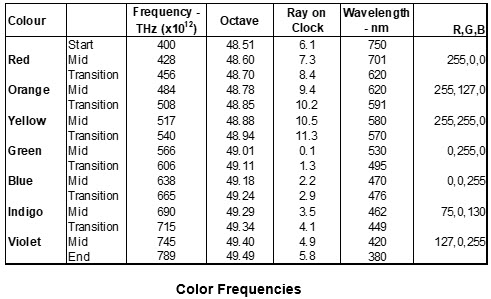The diagram indicates the resonant alignments between planetary, sound and visible light / color frequencies.

The light spectrum doesn’t divide up into sharply defined colors, more a gradation of color.  Color is somewhat subjective.  Conventionally the spectrum is divided into 7 colors, the colors of the rainbow.  In some cases it is divided into 6 or into 12 discrete colors.

The planetary orbital periods appear to be scattered somewhat randomly around the spiral chart above.  However there is order in their alignment with each other.  This is captured separately in the graph below.  Here the time taken for each planet to orbit the sun is plotted to illustrate their relationships one to another.  This includes the asteroid belt as representing a planetary position (Maldek) and Pluto, but does not go out beyond Pluto.  The planetary frequencies follow the very same pattern except that the graphs slope downwards instead of upwards towards the right.

Here we see that planetary periods (and frequencies) change outwards from planet to planet at a reasonably uniform rate.  The average pattern in question is actually that of a spiral expanding outwards at an expansion rate of √5 = 2.236… per cycle of rotation.  There is a pattern but what it means specifically is open to question.

In addition to the planetary orbital periods around the sun there is also a natural frequency associated with the rotation of planets around their own axes to form ‘days’.  These are known.  The associated ‘daily’ as opposed to ‘yearly’ frequencies can also be analysed and graphed in similar fashion.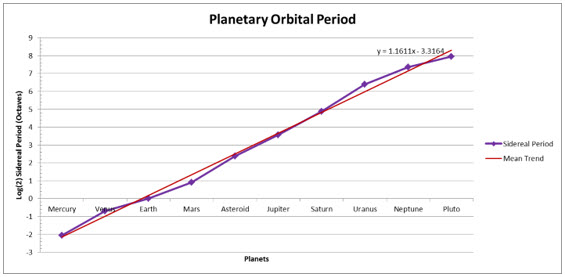## Harmonic Relation between Proportion in Architecture and Sound and Light

Proportion has always been a significant factor in architecture.  Classical architecture used very specific proportions in the design of temples, public buildings, etc.  Some of these proportions or ratios used in Greek temples are illustrated in the diagram over.  These proportions are based on the Pythagorean ratios between powers of 2 and of 3 we have already seen above.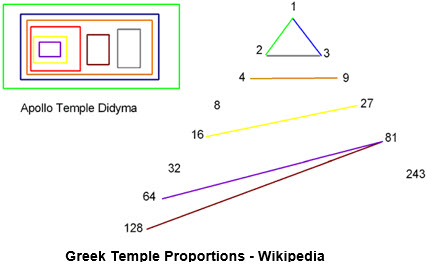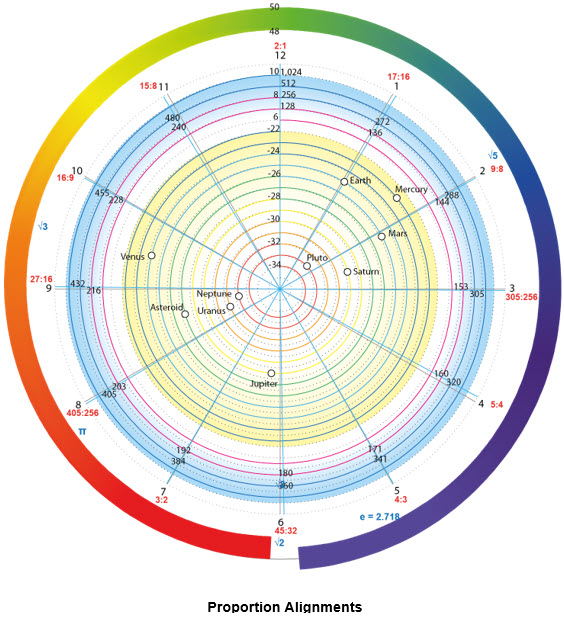Such proportions can be aligned with the frequencies in the octave diagrams.  The clock rays are associated with proportions as we have seen with the music scales above.  The alignment is with the rays themselves and not just with the sound frequencies.  So the proportion alignments are universal and relevant to all frequencies.  The proposed Krystal proportion alignments are illustrated on the octave chart above.  These are the red ratios near the clock numbers.  Some additional numbers of mathematical significance are also shown in blue at their octave locations around the edge.  These include √2, √3, √5, e (2.718..) and π.

This facilitates the identification of resonant alignments between specific proportions and sound, light and planetary frequencies.  It would be interesting to study these resonances for classical temple structures, where such alignments most likely were incorporated in the designs.

## Harmonic Relation between Planetary Alignments and Sound and Light

The type of analysis of planetary alignments that is done in such as astrology can also be used to study relationships with light and sound harmonics.  It can be interesting to look more closely at the significance of such as trine, square, etc. circular alignments in the context of spiral structures.

## 24 Ray Structure

In addition to the Allureah Rashatan code ray positions we can also have 12 additional fire chamber rays interleaved between these positions to form a 24 ray clock.  This is illustrated in the diagram below.  This picks up some additional planetary alignments.

The additional fire chamber positions don’t reveal any particularly interesting numbers.  Also the associated ratios are not very tidy.  All the key numbers and ratios are picked up by the basic 12 ray positions that align with the Allureah Rashatan code.  The most powerful alignments are provided by the octave spiral and its associated 12 ray clock.